U.S. Department of Transportation
1200 New Jersey Avenue, SE
Washington, DC 20590
202-366-4000

Federal Highway Administration Research and Technology
Coordinating, Developing, and Delivering Highway Transportation InnovationsThis report is an archived publication and may contain dated technical, contact, and link information
 Federal Highway Administration > Publications > Research Publications > Hydraulics Publications > 99156 > Enhanced Abutment Scour Studies for Compound Channels
 Publication Number: FHWA-RD-99-156 Date: August 2004

# Enhanced Abutment Scour Studies for Compound Channels

## LIST OF SYMBOLS

a = blocked flow area by abutment

A = unobstructed flow area in approach channel

Af1 = blocked flow area in approach-channel floodplain

b = bridge pier width

Bf = width of floodplain in compound channel

Bm = width of main channel in compound channel

Bm1 = width of approach main channel

Bm2 = width of main channel in contracted section

Cn = units conversion coefficient used in equation 23

C0 = best-fit constant in proposed scour formula

Cr = best-fit coefficient in proposed scour formula

CLB = live-bed scour coefficient

d50 = median diameter of sediment

ds = local scour depth

dsc = theoretical long contraction scour depth

dst = unsteady scour depth at any time t

F0 = approach value of densimetric grain Froude number in Lim scour formula

F1 = approach-flow Froude number

Fab = Froude number adjacent to abutment face

k-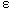= k-epsilon refers to a numerical modeling technique that uses the ratio of turbulent kinetic energy squared to turbulent energy dissipation rate to define the eddy viscosity term for computations

kf = spiral-flow adjustment factor in Maryland scour formula

ks = equivalent sand-grain roughness

kv = velocity adjustment factor in Maryland scour formula

K1 = geometric shape factor for abutment in Froehlich scour formula

K2 = embankment skewness factor in Froehlich scour formula

Ks* = abutment shape factor in Melville scour formula

KG = channel geometric factor in Melville scour formula

KI = flow-intensity factor in Melville scour formula

KST = spill-through abutment shape factor for scour formula in present study

K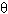* = abutment alignment factor in Melville scour formula

La = abutment/embankment length

M = discharge distribution factor in approach section = unblocked Q over total Q
= discharge contraction ratio for a bankline abutment

n = Manning's n

nfp = Manning's n in floodplain of compound channel

nmc = Manning's n in main channel of compound channel

nK = Manning's n calculated from Keulegan's equation

Ns = sediment number (equivalent to densimetric grain Froude number)

Nsc = critical value of sediment number

q1 = flow rate per unit width in approach section in Maryland scour formula

q2 = flow rate per unit width in contracted section in Maryland scour formula

qbv = volumetric sediment discharge per unit width

qf0 = flow rate per unit width in floodplain at normal depth (unconstricted)

qf0c = critical flow rate per unit width in floodplain at normal depth (unconstricted)

qf1 = flow rate per unit width in the approach floodplain (constricted)

qf2 = flow rate per unit width in the contracted floodplain

qm1 = flow rate per unit width in the approach main channel at the beginning of scour

qm2 = flow rate per unit width in the contracted section in the main channel

Q = total discharge in compound channel

Qf1 = discharge in the approach floodplain

Qm0 = discharge in main channel for uniform flow in a compound channel

Qm1 = discharge in the approach main channel

Qm2 = discharge in the contracted main channel

Qobst1 = obstructed discharge in the approach section

SG = specific gravity of sediment

t = time since beginning of scour

te = time to reach equilibrium scour depth

u*1 = approach value of shear velocity = (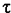1/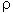)0.5

u*c = critical value of shear velocity = (1/)0.5

Vab = maximum resultant velocity near the upstream corner of the abutment face

Vc = critical velocity for initiation of motion

Vf0 = floodplain velocity at normal depth

Vf0c = critical velocity for unconstricted floodplain depth of uniform flow

Vf1 = bridge approach velocity in floodplain of compound channel

Vf2 = floodplain velocity in the contracted floodplain after scour

Vm0c = critical velocity for the unconstricted depth in the main channel

Vm1 = bridge approach velocity in main channel of compound channel

Vm2c = critical velocity in the main channel at the contracted section at equilibrium scour

Vx = mean contraction velocity in GKY scour formula

VR = resultant velocity adjacent to tip of abutment in GKY scour formula

V1 = bridge approach velocity in rectangular channel

y = generic symbol for depth of flow in main channel or floodplain for calculating critical velocity

yab = depth near the upstream corner of the abutment face

yf0 = normal depth in floodplain

yf1 = bridge approach depth in floodplain of compound channel, including backwater

yftw = tailwater depth in floodplain

ym0 = normal depth in main channel

ym1 = bridge approach depth in main channel of compound channel, including backwater

ym2 = depth of flow in main channel in contracted section at equilibrium scour

ysc = total depth of flow after scour, including the contraction scour depth only (Maryland formula)

y1 = bridge approach depth in rectangular channel

y2 = total flow depth in contracted section after scour (Maryland formula)

y' = vertical coordinate measuring distance above the main-channel bed

y'/ym= ratio of distance above the main-channel bed to depth of the flow in the main channel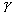= specific weight of fluids = specific weight of sediment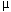f = general discharge per unit width contraction ratio for the floodplain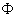= ratio of boundary shear force to streamwise component of weight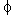= angle of repose of sediment= fluid densitys = sediment density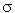= ratio of Manning's n in the main channel with compound-channel flow to the value for the flow in the main channel aloneg = geometric standard deviation of sediment grain size distributionc = critical shear stress for initiation of sediment motion0 = average bed shear stress in uniform flow1 = bed shear stress in approach flow* = Shields' parameter,/[(s -) d50]*c = critical value of Shields' parameter,c/[(s -) d50]*1 = Shields' parameter in approach flow section*2 = Shields' parameter in contracted flow section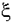= independent dimensionless ratio in proposed scour formula = qf1/Mqf0c